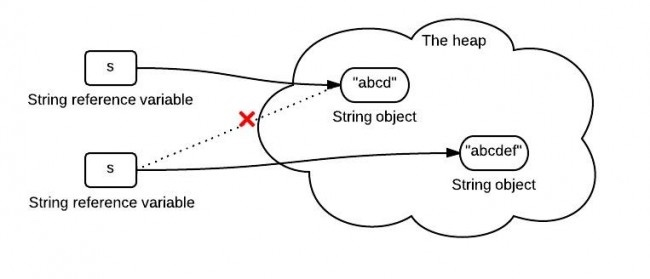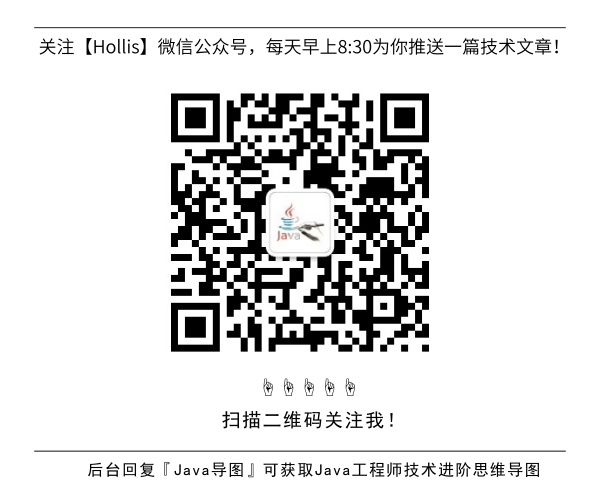# 为什么阿里巴巴不建议在for循环中使用”+”进行字符串拼接

GitHub 19k Star 的Java工程师成神之路，不来了解一下吗！

Java 7 源码学习系列（一）——String

Java中的Switch对整型、字符型、字符串型的具体实现细节

### 字符串拼接

``````String s = "abcd";
s = s.concat("ef");
``````s中保存的是一个重新创建出来的String对象的引用。

``````String wechat = "Hollis";
String introduce = "每日更新Java相关技术文章";
String hollis = wechat + "," + introduce;
``````

concat

``````String wechat = "Hollis";
String introduce = "每日更新Java相关技术文章";
String hollis = wechat.concat(",").concat(introduce);
``````

StringBuffer

``````StringBuffer wechat = new StringBuffer("Hollis");
String introduce = "每日更新Java相关技术文章";
StringBuffer hollis = wechat.append(",").append(introduce);
``````

StringBuilder

``````StringBuilder wechat = new StringBuilder("Hollis");
String introduce = "每日更新Java相关技术文章";
StringBuilder hollis = wechat.append(",").append(introduce);
``````

StringUtils.join

``````String wechat = "Hollis";
String introduce = "每日更新Java相关技术文章";
System.out.println(StringUtils.join(wechat, ",", introduce));
``````

``````String []list  ={"Hollis","每日更新Java相关技术文章"};
String result= StringUtils.join(list,",");
System.out.println(result);
//结果：Hollis,每日更新Java相关技术文章
``````(阿里巴巴Java开发手册中关于字符串拼接的规约)

### 使用`+`拼接字符串的实现原理

``````String wechat = "Hollis";
String introduce = "每日更新Java相关技术文章";
String hollis = wechat + "," + introduce;
``````

``````String wechat = "Hollis";
String introduce = "\u6BCF\u65E5\u66F4\u65B0Java\u76F8\u5173\u6280\u672F\u6587\u7AE0";//每日更新Java相关技术文章
String hollis = (new StringBuilder()).append(wechat).append(",").append(introduce).toString();
``````

### concat是如何实现的

``````public String concat(String str) {
int otherLen = str.length();
if (otherLen == 0) {
return this;
}
int len = value.length;
char buf[] = Arrays.copyOf(value, len + otherLen);
str.getChars(buf, len);
return new String(buf, true);
}
``````

### StringBuffer和StringBuilder

`String`类类似，`StringBuilder`类也封装了一个字符数组，定义如下：

``````char[] value;
``````

`String`不同的是，它并不是`final`的，所以他是可以修改的。另外，与`String`不同，字符数组中不一定所有位置都已经被使用，它有一个实例变量，表示数组中已经使用的字符个数，定义如下：

``````int count;
``````

``````public StringBuilder append(String str) {
super.append(str);
return this;
}
``````

``````public AbstractStringBuilder append(String str) {
if (str == null)
return appendNull();
int len = str.length();
ensureCapacityInternal(count + len);
str.getChars(0, len, value, count);
count += len;
return this;
}
``````

append会直接拷贝字符到内部的字符数组中，如果字符数组长度不够，会进行扩展。

`StringBuffer``StringBuilder`类似，最大的区别就是`StringBuffer`是线程安全的，看一下`StringBuffer``append`方法。

``````public synchronized StringBuffer append(String str) {
toStringCache = null;
super.append(str);
return this;
}
``````

### StringUtils.join是如何实现的

``````public static String join(final Object[] array, String separator, final int startIndex, final int endIndex) {
if (array == null) {
return null;
}
if (separator == null) {
separator = EMPTY;
}

// endIndex - startIndex > 0:   Len = NofStrings *(len(firstString) + len(separator))
//           (Assuming that all Strings are roughly equally long)
final int noOfItems = endIndex - startIndex;
if (noOfItems <= 0) {
return EMPTY;
}

final StringBuilder buf = new StringBuilder(noOfItems * 16);

for (int i = startIndex; i < endIndex; i++) {
if (i > startIndex) {
buf.append(separator);
}
if (array[i] != null) {
buf.append(array[i]);
}
}
return buf.toString();
}
``````

### 效率比较

``````long t1 = System.currentTimeMillis();
//这里是初始字符串定义
for (int i = 0; i < 50000; i++) {
//这里是字符串拼接代码
}
long t2 = System.currentTimeMillis();
System.out.println("cost:" + (t2 - t1));
``````

``````+ cost:5119
StringBuilder cost:3
StringBuffer cost:4
concat cost:3623
StringUtils.join cost:25726
``````

`StringBuilder`<`StringBuffer`<`concat`<`+`<`StringUtils.join`

`StringBuffer``StringBuilder`的基础上，做了同步处理，所以在耗时上会相对多一些。

StringUtils.join也是使用了StringBuilder，并且其中还是有很多其他操作，所以耗时较长，这个也容易理解。其实StringUtils.join更擅长处理字符串数组或者列表的拼接。

``````long t1 = System.currentTimeMillis();
String str = "hollis";
for (int i = 0; i < 50000; i++) {
String s = String.valueOf(i);
str += s;
}
long t2 = System.currentTimeMillis();
System.out.println("+ cost:" + (t2 - t1));
``````

``````long t1 = System.currentTimeMillis();
String str = "hollis";
for(int i = 0; i < 50000; i++)
{
String s = String.valueOf(i);
str = (new StringBuilder()).append(str).append(s).toString();
}

long t2 = System.currentTimeMillis();
System.out.println((new StringBuilder()).append("+ cost:").append(t2 - t1).toString());
``````

### 总结

1、如果不是在循环体中进行字符串拼接的话，直接使用`+`就好了。

2、如果在并发场景中进行字符串拼接的话，要使用`StringBuffer`来代替`StringBuilder`

(全文完)## HollisChuang's Blog

• 微信咨询
• 去评论
• 回顶
###### 回顶部